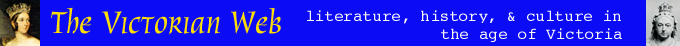## On Maxwell and Maxwellian views of charge and current

#### Diane Greco, M. A., Massachusetts Institute of Technologyhe desire to connect the disparate behaviors of imponderables characterized much physics in the nineteenth century, both on the Continent and in Britain. But imponderables themselves did not persist for very long in the hard-nosed climate of the nineteenth-century. Based on a program of mechanical explanation, the physics of imponderables eventually came to be re-defined, in terms of a unifying concept of energy to which all imponderable phenomena could ultimately be reduced.

By the end of the century, James Clerk Maxwell's derivation of general equations describing the behavior of the electromagnetic field was thought have almost completely fulfilled this demand for a unified physics. The need for a field -- initially a mechanical agency for transporting energy with finite velocity -- arose with the realization, pioneered by H. C. Żrsted and Michael Faraday, that electrical and magnetic phenomena could not be adequately explained as the result of forces exerted between elements of imponderables (central forces). Although Maxwell's model of the field provided a way to understand electromagnetic phenomena from a mechanical point of view, his mathematical structure of the field survived the late nineteenth century rejection of the mechanistic world view (in favor of electrodynamics) and became the basis of later elaborations developed by Heinrich Hertz and others.

In contrast to the mechanical ideal of physics so strongly characteristic of the early nineteenth century, Maxwell's work supported the rise of a more energy-centered physics -- electrodynamics. To be dynamical in the sense used in the mid- to late nineteenth century, a theory need only provide expressions for kinetic and potential energy, expressions that one may deploy in what are known as Lagrange's equations. Lagrange's equations are a set of second-order differential equations that describe a system of particles; the equations relate the kinetic energy of the system to a set of generalized coordinates as this energy changes over time (generalized velocities). But, as Buchwald points out, these coordinates and velocities "need not directly represent an actual mechanical state." For this reason, Maxwell's use of Lagrange's equations rendered mechanical models essentially superfluous. Instead of seeking a complete, tangible realization of the material microstructure thought by many to support electromagnetic phenomena, Maxwellian theory sought unity by means of a set of field equations linked with Hamilton's principle.

Roughly, Hamilton's principle asserts that potential and kinetic energies tend to equalize during a motion. Therefore, Hamilton's principle requires that the path actually taken by any physical system between two states at specified times and with appropriate constants (for the medium of propagation) at these times must be such that the potential and kinetic energy of the system are an extremum -- their difference, integrated over the time interval, equals zero. In Maxwell's hands, Hamilton's principle became one important key to the transformation from a mechanical interpretation of electromagnetic phenomena to a dynamic one. In this way, Maxwell's use of the principle neatly aligns his work with a central characteristic of nineteenth-century physics in general -- the concept of energy as a unifying foundation for all interactions of imponderables.

Maxwellian theory has one deep feature that distinguishes it from electromagnetism after the electron: Maxwellian theory assumes that applying Hamilton's principle to suitably chosen field energy densities (with appropriate medium constants) is all that is necessary to obtain satisfactory mathematical expressions of electromagnetic phenomena. That is to say, if one adopts Hamilton's principle as the fundamental formula of electromagnetism, every problem in field theory reduces to finding an appropriate expression for the field's potential and kinetic energies. As a result, where modern theory introduces the electron, Maxwellian theory introduced new forms of energy.

Today, Maxwell's equations are foundational; even Albert Einstein's revolutionary theory of special relativity took Maxwell's equations as uniquely invariable between different frames of reference. To put a finer point on the difference between Maxwellian physics and today's understanding of electricity and magnetism, Jed Buchwald's summary is worth quoting in full: For the Maxwellians, the world was fundamentally a continuum, and the laws which governed it had to be expressed in an appropriate mathematical form. [É] The goal of the theory was a general set of equations containing variables whose values were defined at every point. Phenomena were to be generated by manipulating functions of these variables -- in particular, energy functions. In practice this meant that the Maxwellians were willing to alter what modern theory considers to be basic equations and were unconcerned with the factors modern theory uses to avoid modifying the basic equations, namely, material microstructure.

Maxwellian charge and current

In modern electromagnetic theory, charge is the source of electric fields, and current is the source of the magnetic fields. In Maxwellian theory, by contrast, charge is produced by the electric field as a result of what was known as "displacement" (of which more anon). As Maxwell and his contemporaries understood it, charge did not provoke an image of a substance; it was not a "stuff."

Moreover, current, in the usual sense of the rate of change of charge over time, was related only indirectly to the magnetic field at this time. As historian Jed Buchwald has demonstrated, in Maxwellian theory "a current is not a substance to be acted upon; it is merely a condition to be changed." That is, current was not considered as a flow of charged particles, but as a series of chargings and dischargings. Maxwellians interpreted these chargings and dischargings as the growth and decay of "displacement," that is, the shift in location of some "incompressible substance" (such as the ether) whose very shifting endows it with a potential energy. If nothing happens to change this potential energy, Maxwellians reason that it will simply dissipate, lost as material heat. This loss results in an inequality of displacement values (between say, one charged surface and another, uncharged one) that engenders to a discontinuity between the two regions. The magnitude of this discontinuity is the charge.